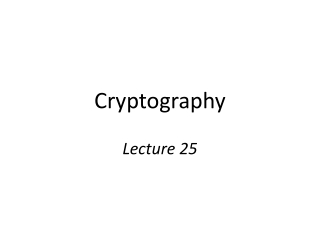DownloadDownload PresentationCryptography

# Cryptography

Télécharger la présentation## Cryptography

- - - - - - - - - - - - - - - - - - - - - - - - - - - E N D - - - - - - - - - - - - - - - - - - - - - - - - - - -
##### Presentation Transcript

1. Cryptography Lecture 25

2. Public-key encryption pk pk c pk pk, sk cEncpk(m) m = Decsk(c)

3. Public-key encryption • A public-key encryption scheme is composed of three PPT algorithms: • Gen: key-generation algorithm that on input 1n outputs pk, sk • Enc: encryption algorithm that on input pk and a message m outputs a ciphertext c • Dec: decryption algorithm that on input sk and a ciphertext c outputs a message m or an error  For all m and pk, sk output by Gen,Decsk(Encpk(m)) = m

4. CPA-security • Fix a public-key encryption scheme  and an adversary A • Define experiment PubK-CPAA,(n): • Run Gen(1n) to get keys pk, sk • Give pk to A, who outputs (m0, m1) of same length • Choose uniform b  {0,1} and compute the ciphertext c  Encpk(mb); give c to A • A outputs a guess b’, and the experiment evaluates to 1 if b’=b

5. CPA-security • Public-key encryption scheme  is CPA-secure if for all PPT adversaries A:Pr[PubK-CPAA,(n) = 1] ≤ ½ + negl(n)

6. Notes on the definition • No encryption oracle?! • Encryption oracle redundant in public-key setting  No perfectly secret public-key encryption • No deterministic public-key encryption scheme can be CPA-secure • CPA-security implies security for encrypting multiple messages as in the private-key case

7. Chosen-ciphertext attacks c pk pk, sk cEncpk(m) c’ m’

8. Chosen-ciphertext attacks • Chosen-ciphertext attacks are arguably even a greater concern in the public-key setting • Attacker might be a legitimate sender • Easier for attacker to obtain full decryptions of ciphertexts of its choice • Related concern: malleability • I.e., given a ciphertext c that is the encryption of an unknown message m, might be possible to produce ciphertext c’ that decrypts to a related message m’ • This is also undesirable in the public-key setting

9. Chosen-ciphertext attacks • Can define CCA-security for public-key encryption by analogy to the definition for private-key encryption • See book for details

10. Hybrid encryption Decryption done in the obvious way Enc’ m ciphertext “encapsulated key” Enc k pk The functionality of public-key encryption at the (asymptotic) efficiency of private-key encryption!

11. Security of hybrid encryption • Let  be the public-key component, and ’ the private-key component; let hy denote their combination • If  is a CPA-secure public-key scheme, and ’ is a CPA-secure private-key scheme, then hyis a CPA-secure public-key scheme • Similarly for CCA-security

12. KEM/DEM paradigm • For hybrid encryption, something weaker than public key encryption would suffice • Sufficient to have an “encapsulation algorithm” that takes a public key and outputs a ciphertext/key pair (c, k) • Correctness: k is recoverable from c given sk • Security: k is indistinguishable from uniform given pk and c • This can lead to more-efficient constructions

13. Dlog-based PKE

14. Diffie-Hellman key exchange G, q, g, h1 h2 • (G, q, g)  G(1n) • x  ℤq • h1 = gx • y  ℤq • h2 = gy c2 = k · m k = (h1)y k = (h2)x m = c2/k

15. El Gamal encryption Public key G, q, g, h1 h2, h1y · m h2 • (G, q, g)  G(1n) • x  ℤq • h1 = gx • y  ℤq • h2 = gy c2 = k · m k = (h1)y k = (h2)x m = c2/k

16. El Gamal encryption • Gen(1n) • Run G(1n) to obtain G, q, g. Choose uniform xℤq. The public key is (G, q, g, gx) and the private key is x • Encpk(m), where pk = (G, q, g, h) and mG • Choose uniform y ℤq.The ciphertext isgy, hy·m • Decsk(c1, c2) • Output c2/c1x

17. Security? • If the DDH assumption is hard for G, then the El Gamal encryption scheme is CPA-secure • Follows from security of Diffie-Hellman key exchange, or can be proved directly • (Discrete-logarithm assumption alone is not enough here)

18. In practice… • Parameters G, q, g are standardized and shared • Inconvenient to treat message as group element • Use key derivation to derive a key k instead, and use k to encrypt the message • I.e., ciphertext is gy, Enc’k(m),where k = H(hy) • Can be analyzed using KEM/DEM paradigm

19. Chosen-ciphertext attacks? • El Gamal encryption is not secure against chosen-ciphertext attacks • Follows from the fact that it is malleable • Given ciphertext c1, c2, transform it to obtain the ciphertext c1, c’2 = c1,  · c2 for arbitrary  • Since c1, c2 = gy, hy · m, we have c1, c’2 = gy, hy · (m) • I.e., encryption of m becomes an encryption of m!

20. Attack! (Assume 2  G  ℤ*p) G, q, g, h c1, c2 c1, 2 ·c2 First bid: mSecond bid: 2m

21. Chosen-ciphertext security • Use key derivation coupled with CCA-secure private-key encryption scheme • I.e., ciphertext is gy, Enc’k(m),where k = H(hy) and Enc’ is a CCA-secure scheme • Can be proved CCA-secure under appropriate assumptions, if H is modeled as a random oracle • DHIES/ECIES